# To STOP or not to STOP A question

• Slides: 23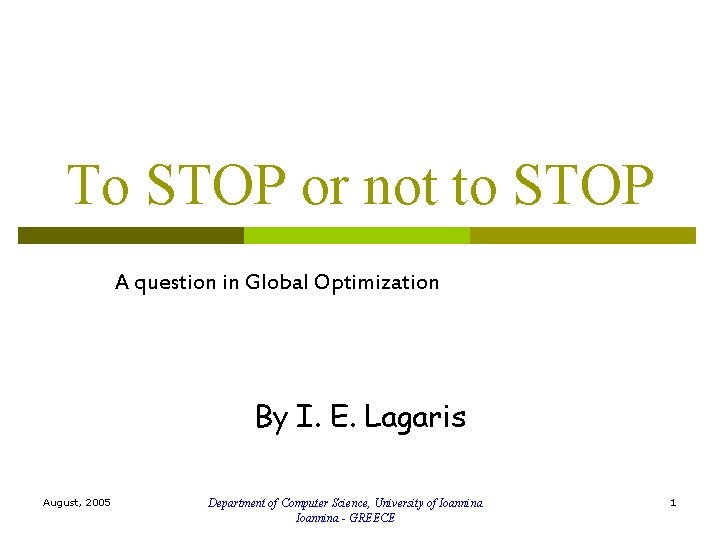To STOP or not to STOP A question in Global Optimization By I. E. Lagaris August, 2005 Department of Computer Science, University of Ioannina - GREECE 1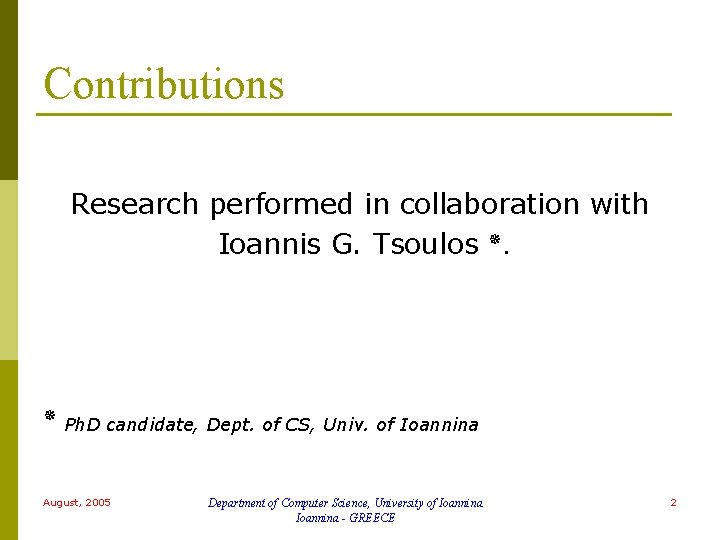Contributions Research performed in collaboration with Ioannis G. Tsoulos . Ph. D candidate, Dept. of CS, Univ. of Ioannina August, 2005 Department of Computer Science, University of Ioannina - GREECE 2Searching for “Local Minima” One-Dimensional Example Exhaustive procedure: From left to right minimization-maximization repetition. August, 2005 Department of Computer Science, University of Ioannina - GREECE 3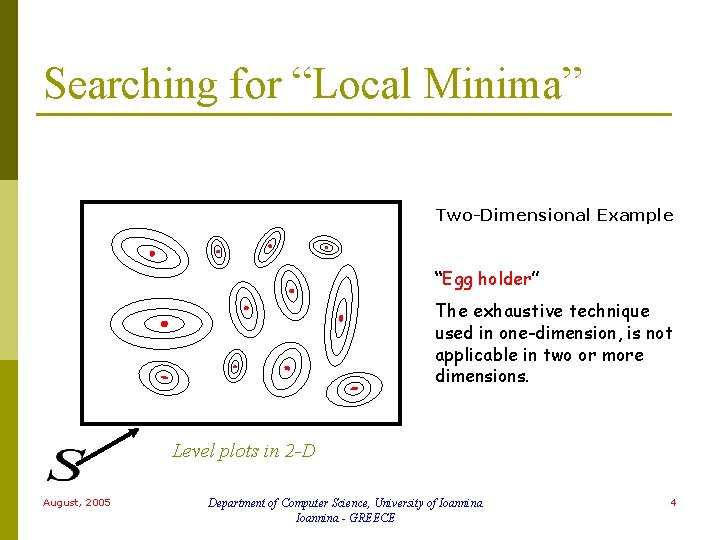Searching for “Local Minima” Two-Dimensional Example “Egg holder” The exhaustive technique used in one-dimension, is not applicable in two or more dimensions. Level plots in 2 -D August, 2005 Department of Computer Science, University of Ioannina - GREECE 4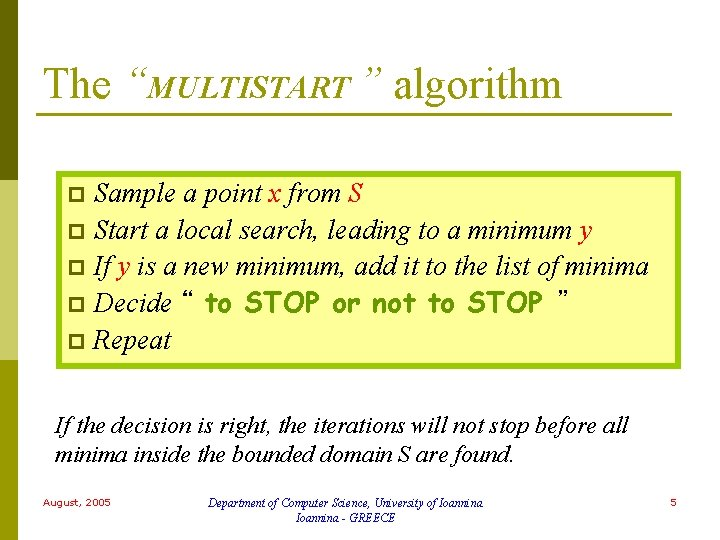The “MULTISTART ” algorithm Sample a point x from S p Start a local search, leading to a minimum y p If y is a new minimum, add it to the list of minima p Decide “ to STOP or not to STOP ” p Repeat p If the decision is right, the iterations will not stop before all minima inside the bounded domain S are found. August, 2005 Department of Computer Science, University of Ioannina - GREECE 5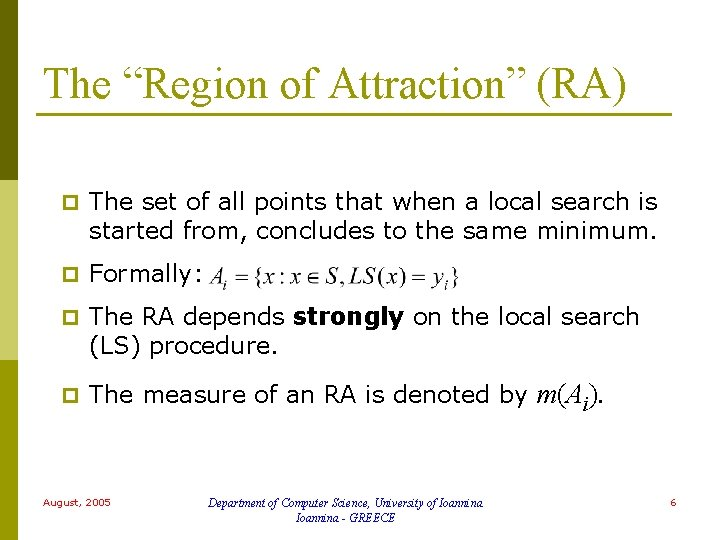The “Region of Attraction” (RA) p The set of all points that when a local search is started from, concludes to the same minimum. p Formally: p The RA depends strongly on the local search (LS) procedure. p The measure of an RA is denoted by August, 2005 Department of Computer Science, University of Ioannina - GREECE m(Ai). 6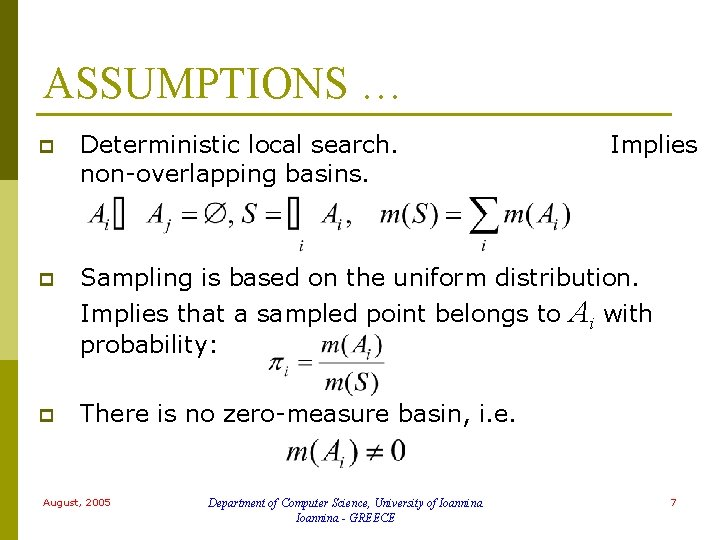ASSUMPTIONS … p Deterministic local search. non-overlapping basins. p Sampling is based on the uniform distribution. Implies that a sampled point belongs to Ai with probability: p There is no zero-measure basin, i. e. August, 2005 Department of Computer Science, University of Ioannina - GREECE Implies 7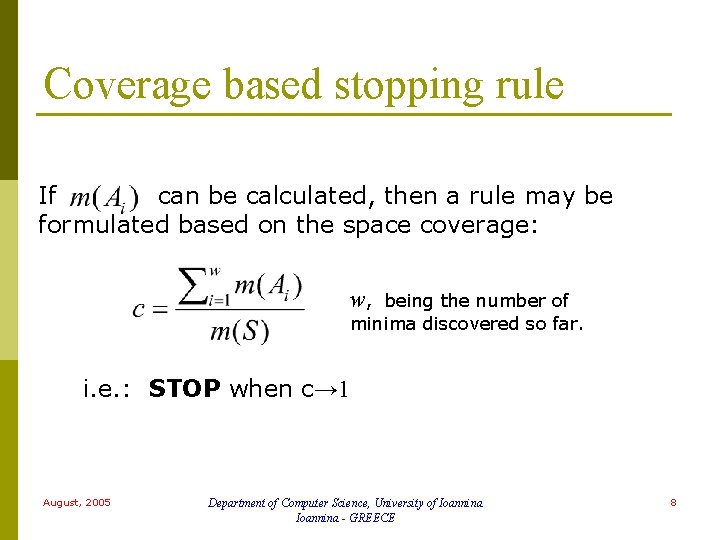Coverage based stopping rule If can be calculated, then a rule may be formulated based on the space coverage: w, being the number of minima discovered so far. i. e. : STOP when c→ 1 August, 2005 Department of Computer Science, University of Ioannina - GREECE 8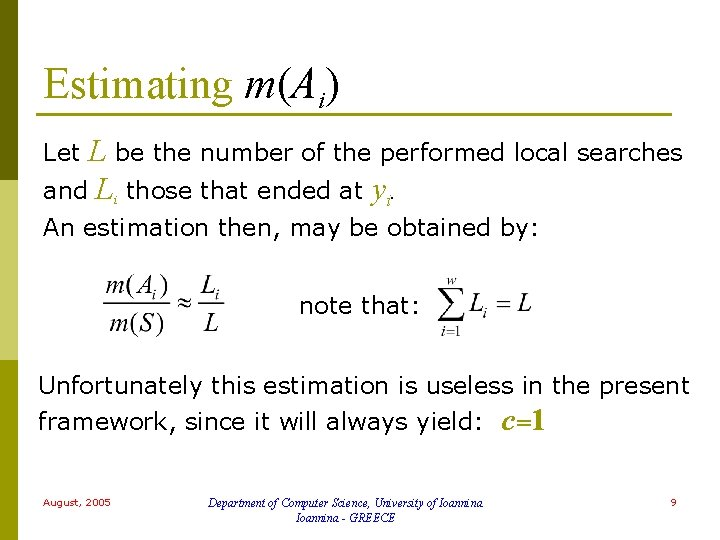Estimating m(Ai) L be the number of the performed local searches and Li those that ended at yi. Let An estimation then, may be obtained by: note that: Unfortunately this estimation is useless in the present framework, since it will always yield: c=1 August, 2005 Department of Computer Science, University of Ioannina - GREECE 9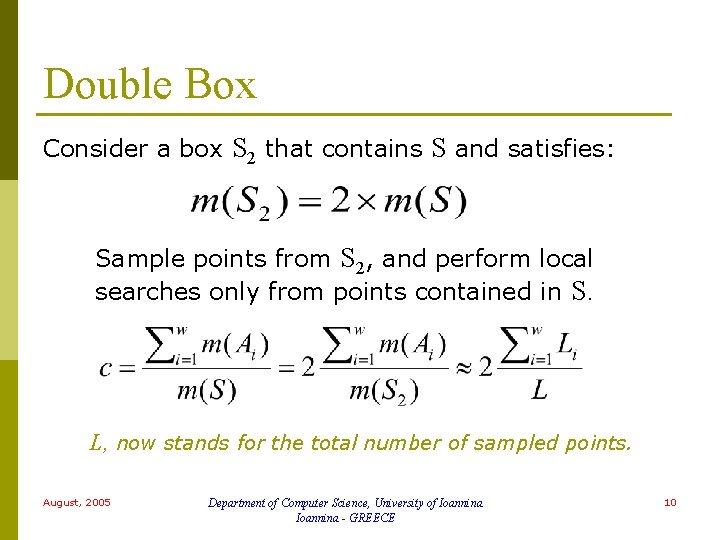Double Box Consider a box S 2 that contains S and satisfies: Sample points from S 2, and perform local searches only from points contained in S. L, now stands for the total number of sampled points. August, 2005 Department of Computer Science, University of Ioannina - GREECE 10Implementation p p Keep sampling from S 2 until N points in S are collected. (N =1 for Multistart) At iteration k, let Mk be the total number of sampled points (k. N of them in S). and → 1 → 0 p STOP if: August, 2005 and Department of Computer Science, University of Ioannina - GREECE last indicates the iteration during which the latest minimum was discovered 11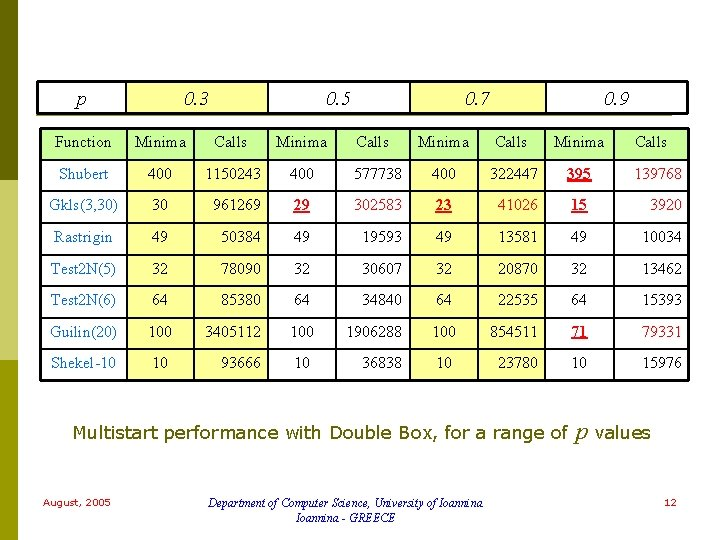p 0. 3 0. 5 Function Minima Calls Minima Shubert 400 1150243 400 Gkls(3, 30) 30 961269 Rastrigin 49 Test 2 N(5) 0. 7 Calls 0. 9 Minima Calls Minima 577738 400 322447 395 139768 29 302583 23 41026 15 3920 50384 49 19593 49 13581 49 10034 32 78090 32 30607 32 20870 32 13462 Test 2 N(6) 64 85380 64 34840 64 22535 64 15393 Guilin(20) 100 3405112 100 1906288 100 854511 71 79331 Shekel-10 10 93666 10 36838 10 23780 10 15976 Multistart performance with Double Box, for a range of August, 2005 Department of Computer Science, University of Ioannina - GREECE Calls p values 12Observables rule This rule relies on the agreement of values of observable (i. e. measurable) quantities, to their expected asymptotic values. p The number of times Li that minimum yi is found, is compared to its expected value. p yi are indexed in order of their appearance. Hence y 1 requires one application of the LS, y 2 requires additional n 2 applications, y 3 additional n 3 … p Let the number of the recovered minima so far be denoted by w. p August, 2005 Department of Computer Science, University of Ioannina - GREECE 13Expectation values The expectation value of the number of times the ith minimum is found, at the time when the wth minimum is recovered for the first time, is recursively given by: An estimation that may be used is: August, 2005 Department of Computer Science, University of Ioannina - GREECE 14Keep trying … Suppose that after having found w minima, there is a number (say K) of consecutive trials without any success, i. e. without finding a new minimum. The expected number of times the ith minimum is found at that moment is given recursively by: August, 2005 Department of Computer Science, University of Ioannina - GREECE 15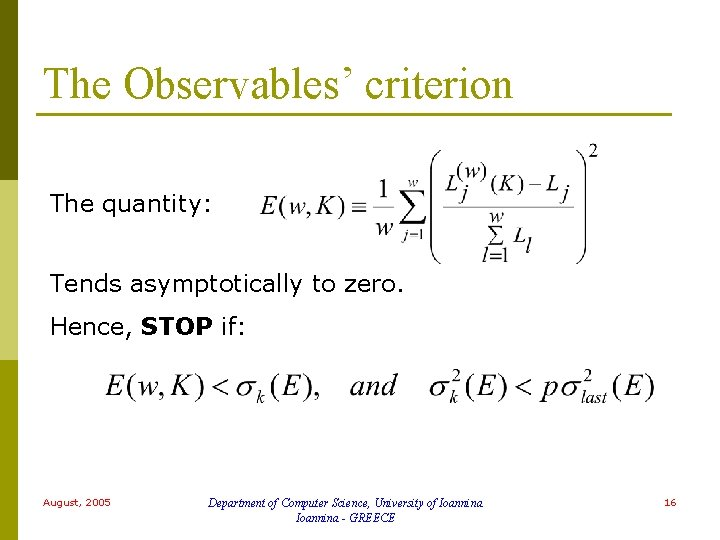The Observables’ criterion The quantity: Tends asymptotically to zero. Hence, STOP if: August, 2005 Department of Computer Science, University of Ioannina - GREECE 16“Expected Minimizers” Rule Based on estimating the number of local minima inside the domain of interest. p The estimation is improving as the algorithm proceeds. p p The key quantity is the probability that l minima are found after m trials. p Calculated recursively. August, 2005 Department of Computer Science, University of Ioannina - GREECE 17Probabilities If stands for the probability to recover then the probability of finding in a single trial, l minima in m trials is given by: Note that: Probability that a new minimum is found other than August, 2005 Probability that one of the first l minima is found again. Department of Computer Science, University of Ioannina - GREECE 18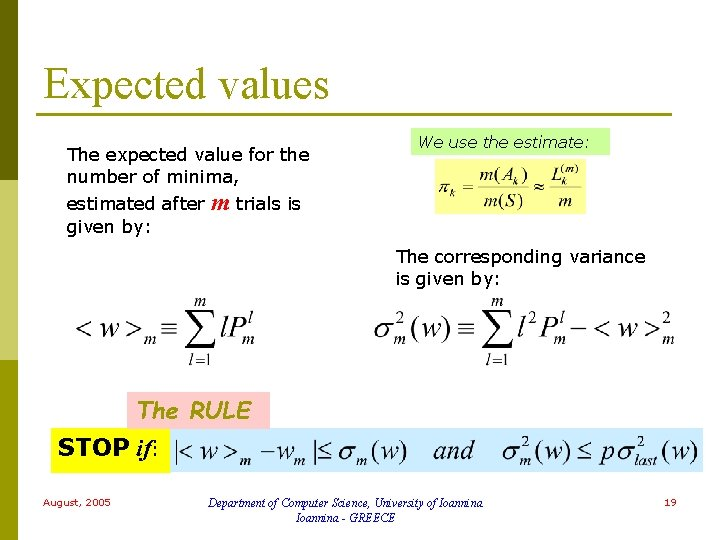Expected values The expected value for the number of minima, estimated after m trials is given by: We use the estimate: The corresponding variance is given by: The RULE STOP if: August, 2005 Department of Computer Science, University of Ioannina - GREECE 19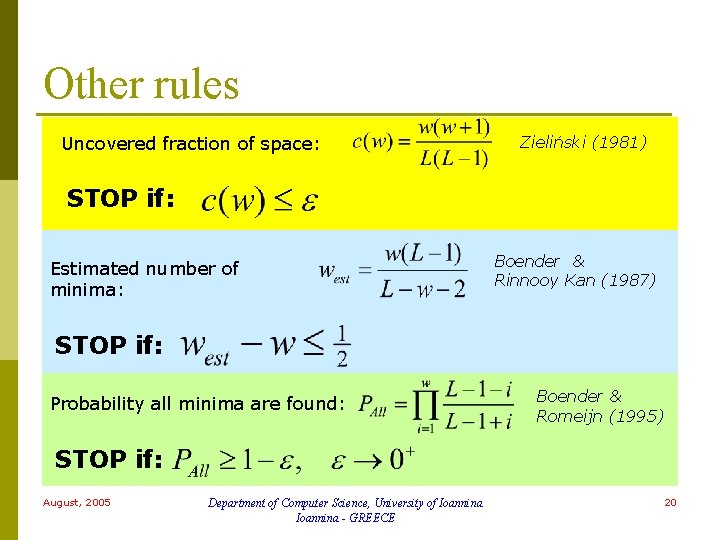Other rules Uncovered fraction of space: Zieliński (1981) STOP if: Estimated number of minima: Boender & Rinnooy Kan (1987) STOP if: Probability all minima are found: Boender & Romeijn (1995) STOP if: August, 2005 Department of Computer Science, University of Ioannina - GREECE 20MULTISTART Uncovered fraction August, 2005 Estimated # of minima Double Box Department of Computer Science, University of Ioannina - GREECE Observables Expected # of minima 21TMLSL Uncovered fraction August, 2005 Estimated # of minima Double Box Department of Computer Science, University of Ioannina - GREECE Observables Expected # of minima 22Conclusions p The new rules improve the performance at least for problems in our benchmark suite. Proper choice of the parameter p, for different methods is important. p Remains to be seen if performance is also boosted in other practical applications. p August, 2005 Department of Computer Science, University of Ioannina - GREECE 23